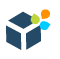DWBI.org Login / Sign Up
New Account?
Recovery
Oracle

# Oracle Analytic Functions

Oracle Analytic Functions compute an aggregate value based on a group of rows. It opens up a whole new way of looking at the data. This article explains how we can unleash the full potential of this.

Analytic functions differ from aggregate functions in the sense that they return multiple rows for each group. The group of rows is called a window and is defined by the analytic clause. For each row, a sliding window of rows is defined. The window determines the range of rows used to perform the calculations for the current row.

Oracle provides many Analytic Functions such as

AVG, CORR, COVAR_POP, COVAR_SAMP, COUNT, CUME_DIST, DENSE_RANK, FIRST, FIRST_VALUE, LAG, LAST, LAST_VALUE, LEAD, MAX, MIN, NTILE, PERCENT_RANK, PERCENTILE_CONT, PERCENTILE_DISC, RANK, RATIO_TO_REPORT, STDDEV, STDDEV_POP, STDDEV_SAMP, SUM, VAR_POP, VAR_SAMP, VARIANCE.

The Syntax of analytic functions:

``````Analytic-Function(Column1,Column2,...)
OVER (
[Query-Partition-Clause]
[Order-By-Clause]
[Windowing-Clause]
)``````

Analytic functions take 0 to 3 arguments.

An Example:

``````SELECT ename, deptno, sal,
SUM( sal )
OVER ( ORDER BY deptno, ename ) AS Running_Total,
SUM( sal )
OVER ( PARTITION BY  deptno
ORDER BY  ename) AS Dept_Total,
ROW_NUMBER()
OVER ( PARTITION BY deptno
ORDER BY ename ) As Sequence_No
FROM emp
ORDER BY deptno, ename;``````

The partition clause makes the SUM(sal) be computed within each department, independent of the other groups. The SUM(sal) is 'reset' as the department changes. The ORDER BY ENAME clause sorts the data within each department by ENAME;

• Query-Partition-Clause

The PARTITION BY clause logically breaks a single result set into N groups, according to the criteria set by the partition expressions. The analytic functions are applied to each group independently, they are reset for each group.

• Order-By-Clause

The ORDER BY clause specifies how the data is sorted within each group (partition). This will definitely affect the output of the analytic function.

• Windowing-Clause

The windowing clause gives us a way to define a sliding or anchored window of data, on which the analytic function will operate, within a group. This clause can be used to have the analytic function compute its value based on any arbitrary sliding or anchored window within a group. The default window is an anchored window that simply starts at the first row of a group and continues to the current row.

Let's look an example with a sliding window within a group and compute the sum of the current row's salary column plus the previous 2 rows in that group. i.e ROW Window clause:

``````SELECT deptno, ename, sal,
SUM( sal )
OVER  (  PARTITION BY  deptno
ORDER BY ename
ROWS 2 PRECEDING  ) AS Sliding_Total
FROM emp
ORDER BY deptno, ename;``````

Now if we look at the Sliding Total value of SMITH it is simply SMITH's salary plus the salary of two preceding rows in the window. [ 800+3000+2975 = 6775 ]

We can set up windows based on two criteria: RANGES of data values or ROWS offset from the current row. It can be said, that the existence of an ORDER BY in an analytic function will add a default window clause of RANGE UNBOUNDED PRECEDING. That says to get all rows in our partition that came before us as specified by the ORDER BY clause.

### ** Solving Top-N Queries **

Suppose we want to find out the top 3 salaried employee of each department:

``````SELECT deptno, ename, sal, ROW_NUMBER()
OVER (
PARTITION BY deptno ORDER BY sal DESC
) Rnk FROM emp;``````

This will give us the employee name and salary with ranks based on descending order of salary for each department or the partition/group . Now to get the top 3 highest paid employees for each dept.

``````SELECT * FROM (
SELECT deptno, ename, sal, ROW_NUMBER()
OVER (
PARTITION BY deptno ORDER BY sal DESC
) Rnk FROM emp
) WHERE Rnk <= 3;``````

The use of a WHERE clause is to get just the first three rows in each partition.

### ** Solving the problem with DENSE_RANK **

If we look carefully the above output we will observe that the salary of SCOTT and FORD of dept 10 are same. So we are indeed missing the 3rd highest salaried employee of dept 20. Here we will use DENSE_RANK function to compute the rank of a row in an ordered group of rows. The ranks are consecutive integers beginning with 1. The DENSE_RANK function does not skip numbers and will assign the same number to those rows with the same value.

The above query now modified as:

``````SELECT * FROM (
SELECT deptno, ename, sal,  DENSE_RANK()
OVER (
PARTITION BY deptno ORDER BY sal DESC
) Rnk FROM emp
)
WHERE Rnk <= 3;``````

and the output is as follows: